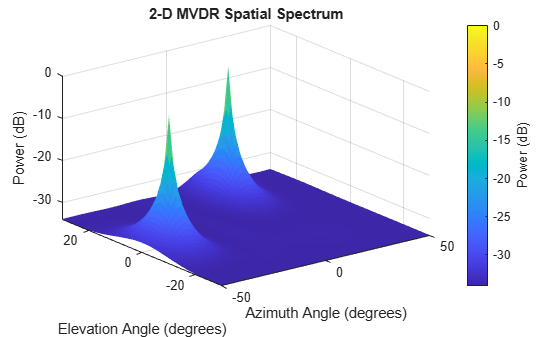# step

System object: phased.MVDREstimator2D
Package: phased

Perform spatial spectrum estimation

## Syntax

```Y = step(H,X) [Y,ANG] = step(H,X) ```

## Description

Note

Starting in R2016b, instead of using the `step` method to perform the operation defined by the System object™, you can call the object with arguments, as if it were a function. For example, ```y = step(obj,x)``` and `y = obj(x)` perform equivalent operations.

`Y = step(H,X)` estimates the spatial spectrum from `X` using the estimator `H`. `X` is a matrix whose columns correspond to channels. `Y` is a matrix representing the magnitude of the estimated 2-D spatial spectrum. The row dimension of `Y` is equal to the number of angles in the `ElevationScanAngles` and the column dimension of `Y` is equal to the number of angles in the `AzimuthScanAngles` property. You can specify the argument, `X`, as single or double precision.

The size of the first dimension of the input matrix can vary to simulate a changing signal length. A size change can occur, for example, in the case of a pulse waveform with variable pulse repetition frequency.

`[Y,ANG] = step(H,X)` returns additional output `ANG` as the signal’s direction of arrival (DOA) when the `DOAOutputPort` property is `true`. `ANG` is a two-row matrix where the first row represents estimated azimuth and the second row represents estimated elevation (in degrees).

Note

The object performs an initialization the first time the object is executed. This initialization locks nontunable properties and input specifications, such as dimensions, complexity, and data type of the input data. If you change a nontunable property or an input specification, the System object issues an error. To change nontunable properties or inputs, you must first call the `release` method to unlock the object.

## Examples

expand all

Estimate the DOAs of two signals received by a 50-element URA with a rectangular lattice. The antenna operating frequency is 150 MHz. The actual direction of the first signal is -37° in azimuth and 0° in elevation. The direction of the second signal is 17° in azimuth and 20° in elevation.

Create signals sampled at 8 kHz.

```fc = 150e6; fs = 8000; t = (0:1/fs:1).'; x1 = cos(2*pi*t*300); x2 = cos(2*pi*t*400); array = phased.URA('Size',[5 10],'ElementSpacing',[1 0.6]); array.Element.FrequencyRange = [100e6 300e6]; x = collectPlaneWave(array,[x1 x2],[-37 0;17 20]',fc);```

`noise = 0.1*(randn(size(x))+1i*randn(size(x)));`

Create the MVDR DOA estimator for URA.

```estimator = phased.MVDREstimator2D('SensorArray',array,... 'OperatingFrequency',fc,... 'DOAOutputPort',true,'NumSignals',2,... 'AzimuthScanAngles',-50:50,... 'ElevationScanAngles',-30:30);```

Use the `step` method to the DOA estimates.

`[~,doas] = estimator(x + noise)`
```doas = 2×2 17 -37 20 0 ```

Plot the spectrum.

`plotSpectrum(estimator)`